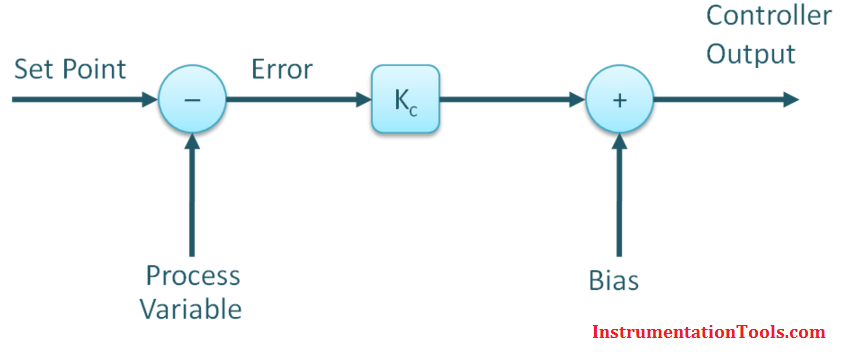# Why Bias used in Proportional Controller ?

The P-Only Algorithm

The P-Only controller computes a CO action every loop sample time T as:

CO = CObias + Kc∙e(t)

Where:
CObias = controller bias or null value
Kc = controller gain, a tuning parameter
e(t) = controller error = SP – PV
SP = set point
PV = process variable

## Understanding Controller Bias

Let’s suppose the P-Only control algorithm shown above is used for speed control in an automobile and CO is the throttle signal adjusting the flow of fuel to the engine.

Let’s also suppose that the speed SP is 70 and the measured PV is also 70 (units can be mph or kph ). Since PV = SP, then e(t) = 0 and the algorithm reduces to:

#### CO = CObias + Kc∙(0) = CObiasIf CObias is zero, then when set point equals measurement, the above equation says that the throttle signal, CO, is also zero. This makes no sense. Clearly if the car is traveling 70 kph, then some baseline flow of fuel is going to the engine.

This baseline value of the CO is called the bias or null value. In this example, CObias is the flow of fuel that, in manual mode, causes the car to travel the set speed of 70 kph.

A P-Only controller bias (sometimes called null value) is assigned a value as part of the controller design and remains fixed once the controller is put in automatic.

### Controller Gain, Kc

The P-Only controller has the advantage of having only one adjustable or tuning parameter, Kc, that defines how active or aggressive the CO will move in response to changes in controller error, e(t).

For a given value of e(t) in the P-Only algorithm above, if Kc is small, then the amount added to CObias is small and the controller response will be slow or sluggish. If Kc is large, then the amount added to CObias is large and the controller response will be fast or aggressive.

Thus, Kc can be adjusted or tuned for each process to make the controller more or less active in its actions when measurement does not equal set point.

Also Read: Why Offset in Proportional Controller ?